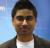# PowerCharts' DragNode doesn't load images

## Recommended Posts

Hi all.

I'm evaluating FusionCharts' PowerCharts to see if they fit my needs, and I tried to use DragNode chart with images. It works ok on my local computer, but when I put the chart on my web server it doesn't load any images. I've tried to put images in all kinds of directories and use all kinds of URLs to refer to them - relative, absolute, full, whatever - and nothing works.

I even looked at the web server request logs and I can see that there are no calls from my IP to any URL that looks something similar to the image files I'm trying to load.

Here is the XML that the DragNode chart reads - I can see it reads it properly because all the items described in it are rendered properly:

```<chart palette="4" xAxisMinValue="0" xAxisMaxValue="100" yAxisMinValue="0" yAxisMaxValue="100" is3D="1" showFormBtn="0" viewMode="1" use3DLighting="1">

<dataset>

<set x="17" y="79" width="52" height="75" name="Previous item" color="FE3233" id="1" labelAlign="TOP" toolText="Just Me" shape="RECTANGLE" imageurl="thumbs/mcf603002/1.jpg" imageAlign="BOTTOM" imageHeight="38" imageWidth="45"/>

<set x="83" y="79" width="52" height="75" name="This item" color="FE6464" id="2" labelAlign="TOP" toolText="Dancing" shape="RECTANGLE" imageurl="thumbs/mcf603002/1.jpg" imageAlign="BOTTOM" imageHeight="38" imageWidth="45"/>

<set x="50" y="50" radius="30" name="Category" color="3432FF" id="3" shape="circle"/>

<set x="21" y="20" width="52" height="52" color="FECC33" id="4" toolText=" " shape="RECTANGLE" imageurl="thumbs/mcf554096/1.jpg" imageAlign="center" imageHeight="38" imageWidth="45"/>

<set x="42" y="20" width="52" height="52" color="FECC33" id="5" toolText=" " shape="RECTANGLE" imageurl="thumbs/mcf495028/1.jpg" imageAlign="center" imageHeight="38" imageWidth="45"/>

<set x="63" y="20" width="52" height="52" color="FECC33" id="6" toolText=" " shape="RECTANGLE" imageurl="thumbs/mcf294638/1.jpg" imageAlign="center" imageHeight="38" imageWidth="45"/>

<set x="84" y="20" width="52" height="52" color="FECC33" id="7" toolText=" " shape="RECTANGLE" imageurl="thumbs/mcf443988/1.jpg" imageAlign="center" imageHeight="38" imageWidth="45"/>

<set x="105" y="20" width="52" height="52" color="FECC33" id="8" toolText=" " shape="RECTANGLE" imageurl="thumbs/mcf571271/1.jpg" imageAlign="center" imageHeight="38" imageWidth="45"/>

</dataset>

<connectors stdThickness="5">

<connector strength="1" label="" from="1" to="3" color="BBBB00" arrowAtStart="0" arrowAtEnd="1"/>

<connector strength="1" label="" from="3" to="2" color="BBBB00" arrowAtStart="0" arrowAtEnd="1"/>

<connector strength="0.3" label="" from="3" to="4" color="FF0000" arrowAtStart="0" arrowAtEnd="1"/>

<connector strength="0.3" label="" from="3" to="5" color="FF0000" arrowAtStart="0" arrowAtEnd="1"/>

<connector strength="0.3" label="" from="3" to="6" color="FF0000" arrowAtStart="0" arrowAtEnd="1"/>

<connector strength="0.3" label="" from="3" to="7" color="FF0000" arrowAtStart="0" arrowAtEnd="1"/>

<connector strength="0.3" label="" from="3" to="8" color="FF0000" arrowAtStart="0" arrowAtEnd="1"/>

</connectors>

</chart>

```

I'm using Firefox 2.0, but I tested it with IE6 and 7 - same behavior.

Any help will be greately appreciated.

Edited by Guest

##### Share on other sites

Yes, they were. I found the problem - I should have also added the imageNode="1" attribute to the sets. I think its not correct and the chart should understand that I want to have an image in the node when I set an imageURL, but that may be just my broken thinking.

anyway - it works, so thanks, and keep up the good work :-)

##### Share on other sites

I realise this topic is quite old but I have a similar issue.  I have a drag node chart using:

imageNode='1' imageurl='/images/icons/org_female.png'

which works fine.  However, in the same chart I use:

imageNode='1' imageurl='page.asp?id=123'

which loads an image (using Response.BinaryWrite) which is secured above the web root.  In this case the image and node text does not display, I just get a blank box.

I use this method elsewhere on standard web pages and it does display the image.

I have tried URL encoding the imageurl but this has no effect.

Any help, much appreciated!

Edited by Guest

##### Share on other sites

JPEG.  Linking to a JPEG directly in the web root works fine.

##### Share on other sites

Not specefied.  It is a generic page to Response.BinaryWrite files stored above the root.  It can be used for any file type.  Does it need to be progessive or non-progressive.